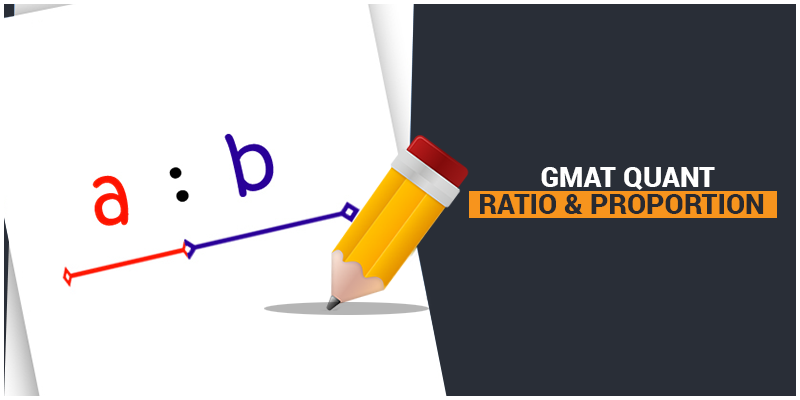# GMAT Quant: Arithmetic – Ratio & ProportionRatio and proportion have always been on your radar since grade school, as it was so tricky that it always confused you to a great extent. Let’s take a quick look at the concepts of Ratio & Proportion and refresh the basics.

What does Ratio mean?

Technically, ratio and fractions are synonymous to one another. So, all the rules of fractions apply to ratio as the well.

What does proportion mean? Are ratios and proportion same?

Ratio and proportion are not same; neither have they held the same meaning. The ratio is a single fraction whereas; proportion sets two different ratios as equal.

Properties of Ratio and Proportion:

• If any two geometric figures are similar to one another, then their sides are said to be in proportion.
• Percentage and probability are special cases of ratios.

Let’s solve some problems and clear the doubts related to this interesting topics.

1. Jacob, Bren and Danny worked in a ratio of 1: 2: 4 hours, respectively. How many hours did Bob work?
1. Together, Jacob, Bren and Danny worked a total of 49 hours.
2. Danny worked 21 hours more than Jacob.

Solution: The ratio of total number of hours Jacob, Bren and Danny worked = 1.

All you need to do is, solve for the value x and you will get the number of hours Bob worked.

Statement A. As given, the total number hours they all together worked = 49

So, according to the ratio; 1x + 2x + 4x = 49

X = 7

So, statement A is sufficient.

Statement B. Danny worked 21 hours more than Jacob.

So, 4x = x + 21

X = 7

X has a unique value. So statement B is also sufficient.

We’ll be glad to help you in your GMAT preparation journey. You can ask for any assistance related to GMAT and MBA from us by calling us at +918884544444. You can write to us at gmat@byjus.com.